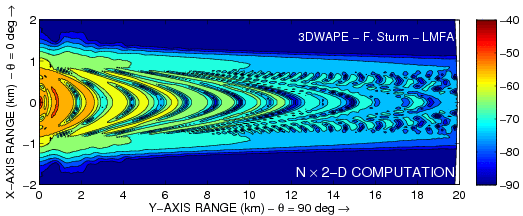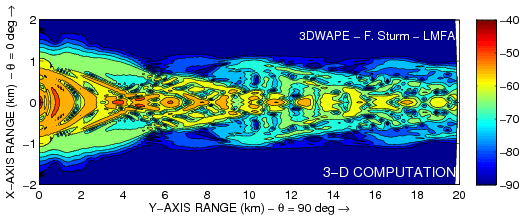# Laboratoire de Mécanique des Fluides et d’Acoustique - UMR 5509

LMFA - UMR 5509
Laboratoire de Mécanique des Fluides et d’Acoustique
Lyon
France

## 3-D Gaussian Canyon - CW at 25 Hz

Sound wave propagation in a three-dimensional gaussian canyon oceanic waveguide.

The 25-Hz CW point source is omnidirectional (source depth of 40 m). Numerical simulations performed using the 3-D parabolic equation based model 3DWAPE.

### Top view of the TL field

Horizontal slices (at a constant depth of 30 m) of the transmission loss field (in dB re 1 m) corresponding to pseudo 3-D (upper subplot) and fully 3-D (lower subplot) PE calculations.### Vertical Slice of the TL field

Vertical slices (at a constant azimuth of 90 deg) of the transmission loss field (in dB re 1 m) corresponding to 2-D (upper subplot) and fully 3-D (lower subplot) PE calculations.